# Title 40

## SECTION 53.23

### 53.23 Test procedures.

§ 53.23 Test procedures.

(a) Range - (1) Technical definition. The nominal minimum and maximum concentrations that a method is capable of measuring.

Note to § 53.23(a)(1):

The nominal range is given as the lower and upper range limits in concentration units, for example, 0-0.5 parts per million (ppm).

(2) Test procedure. Determine and submit a suitable calibration curve, as specified in § 53.21(b), showing the test analyzer's measurement response over at least 95 percent of the required or indicated measurement range.

Note to § 53.23(a)(2):

A single calibration curve for each measurement range for which an FRM or FEM designation is sought will normally suffice.

(b) Noise - (1) Technical definition. Spontaneous, short duration deviations in measurements or measurement signal output, about the mean output, that are not caused by input concentration changes. Measurement noise is determined as the standard deviation of a series of measurements of a constant concentration about the mean and is expressed in concentration units.

(2) Test procedure. (i) Allow sufficient time for the test analyzer to warm up and stabilize. Determine measurement noise at each of two fixed concentrations, first using zero air and then a pollutant test gas concentration as indicated below. The noise limit specification in table B-1 to subpart B of part 53 shall apply to both of these tests.

(ii) For an analyzer with an analog signal output, connect an integrating-type digital meter (DM) suitable for the test analyzer's output and accurate to three significant digits, to determine the analyzer's measurement output signal.

Note to § 53.23(b)(2):

Use of a chart recorder in addition to the DM is optional.

(iii) Measure zero air with the test analyzer for 60 minutes. During this 60-minute interval, record twenty-five (25) test analyzer concentration measurements or DM readings at 2-minute intervals. (See Figure B-2 in appendix A of this subpart.)

(iv) If applicable, convert each DM test reading to concentration units (ppm) or adjust the test readings (if necessary) by reference to the test analyzer's calibration curve as determined in § 53.21(b). Label and record the test measurements or converted DM readings as r1, r2, r3 . . . ri . . . r25.

(v) Calculate measurement noise as the standard deviation, S, as follows: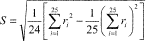Where i indicates the i-th test measurement or DM reading in ppm.

(vi) Let S at 0 ppm be identified as S0; compare S0 to the noise limit specification given in table B-1 to subpart B of part 53.

(vii) Repeat steps in Paragraphs (b)(2)(iii) through (v) of this section using a pollutant test atmosphere concentration of 80 ±5 percent of the URL instead of zero air, and let S at 80 percent of the URL be identified as S80. Compare S80 to the noise limit specification given in table B-1 to subpart B of part 53.

(viii) Both S0 and S80 must be less than or equal to the table B-1 to subpart B of part 53 noise limit specification to pass the test for the noise parameter.

(c) Lower detectable limit - (1) Technical definition. The minimum pollutant concentration that produces a measurement or measurement output signal of at least twice the noise level.

(2) Test procedure. (i) Allow sufficient time for the test analyzer to warm up and stabilize. Measure zero air and record the stable measurement reading in ppm as BZ. (See Figure B-3 in appendix A of this subpart.)

(ii) Generate and measure a pollutant test concentration equal to the value for the lower detectable limit specified in table B-1 to subpart B of part 53.

Note to § 53.23(c)(2):

If necessary, the test concentration may be generated or verified at a higher concentration, then quantitatively and accurately diluted with zero air to the final required test concentration.

(iii) Record the test analyzer's stable measurement reading, in ppm, as BL.

(iv) Determine the lower detectable limit (LDL) test result as LDL = BL − BZ. Compare this LDL value with the noise level, S0, determined in § 53.23(b), for the 0 concentration test atmosphere. LDL must be equal to or higher than 2 × S0 to pass this test.

(d) Interference equivalent - (1) Technical definition. Positive or negative measurement response caused by a substance other than the one being measured.

(2) Test procedure. The test analyzer shall be tested for all substances likely to cause a detectable response. The test analyzer shall be challenged, in turn, with each potential interfering agent (interferent) specified in table B-3 to subpart B of part 53. In the event that there are substances likely to cause a significant interference which have not been specified in table B-3 to subpart B of part 53, these substances shall also be tested, in a manner similar to that for the specified interferents, at a concentration substantially higher than that likely to be found in the ambient air. The interference may be either positive or negative, depending on whether the test analyzer's measurement response is increased or decreased by the presence of the interferent. Interference equivalents shall be determined by mixing each interferent, one at a time, with the pollutant at an interferent test concentration not lower than the test concentration specified in table B-3 to subpart B of part 53 (or as otherwise required for unlisted interferents), and comparing the test analyzer's measurement response to the response caused by the pollutant alone. Known gas-phase reactions that might occur between a listed interferent and the pollutant are designated by footnote 3 in table B-3 to subpart B of part 53. In these cases, the interference equivalent shall be determined without mixing with the pollutant.

(i) Allow sufficient time for warm-up and stabilization of the test analyzer.

(ii) For a candidate method using a prefilter or scrubber device based upon a chemical reaction to derive part of its specificity and which device requires periodic service or maintenance, the test analyzer shall be “conditioned” prior to conducting each interference test series. This requirement includes conditioning for the NO2 converter in chemiluminescence NO/NO2/NOX analyzers and for the ozone scrubber in UV-absorption ozone analyzers. Conditioning is as follows:

(A) Service or perform the indicated maintenance on the scrubber or prefilter device, as if it were due for such maintenance, as directed in the manual referred to in § 53.4(b)(3).

(B) Before testing for each potential interferent, allow the test analyzer to sample through the prefilter or scrubber device a test atmosphere containing the interferent at a concentration not lower than the value specified in table B-3 to subpart B of part 53 (or, for unlisted potential interferents, at a concentration substantially higher than likely to be found in ambient air). Sampling shall be at the normal flow rate and shall be continued for 6 continuous hours prior to the interference test series. Conditioning for all applicable interferents prior to any of the interference tests is permissible. Also permissible is simultaneous conditioning with multiple interferents, provided no interferent reactions are likely to occur in the conditioning system.

(iii) Generate three test atmosphere streams as follows:

(A) Test atmosphere P: Pollutant test concentration.

(B) Test atmosphere I: Interferent test concentration.

(C) Test atmosphere Z: Zero air.

(iv) Adjust the individual flow rates and the pollutant or interferent generators for the three test atmospheres as follows:

(A) The flow rates of test atmospheres I and Z shall be equal.

(B) The concentration of the pollutant in test atmosphere P shall be adjusted such that when P is mixed (diluted) with either test atmosphere I or Z, the resulting concentration of pollutant shall be as specified in table B-3 to subpart B of part 53.

(C) The concentration of the interferent in test atmosphere I shall be adjusted such that when I is mixed (diluted) with test atmosphere P, the resulting concentration of interferent shall be not less than the value specified in table B-3 to subpart B of part 53 (or as otherwise required for unlisted potential interferents).

(D) To minimize concentration errors due to flow rate differences between I and Z, it is recommended that, when possible, the flow rate of P be from 10 to 20 times larger than the flow rates of I and Z.

(v) Mix test atmospheres P and Z by passing the total flow of both atmospheres through a (passive) mixing component to insure complete mixing of the gases.

(vi) Sample and measure the mixture of test atmospheres P and Z with the test analyzer. Allow for a stable measurement reading, and record the reading, in concentration units, as R (see Figure B-3).

(vii) Mix test atmospheres P and I by passing the total flow of both atmospheres through a (passive) mixing component to insure complete mixing of the gases.

(viii) Sample and measure this mixture of P and I with the test analyzer. Record the stable measurement reading, in concentration units, as RI.

(ix) Calculate the interference equivalent (IE) test result as:

IE = RI−R. IE must be within the limits (inclusive) specified in table B-1 to subpart B of part 53 for each interferent tested to pass the interference equivalent test.

(x) Follow steps (iii) through (ix) of this section, in turn, to determine the interference equivalent for each listed interferent as well as for any other potential interferents identified.

(xi) For those potential interferents which cannot be mixed with the pollutant, as indicated by footnote (3) in table B-3 to subpart B of part 53, adjust the concentration of test atmosphere I to the specified value without being mixed or diluted by the pollutant test atmosphere. Determine IE as follows:

(A) Sample and measure test atmosphere Z (zero air). Allow for a stable measurement reading and record the reading, in concentration units, as R.

(B) Sample and measure the interferent test atmosphere I. If the test analyzer is not capable of negative readings, adjust the analyzer (not the recorder) to give an offset zero. Record the stable reading in concentration units as RI, extrapolating the calibration curve, if necessary, to represent negative readings.

(C) Calculate IE = RI − R. IE must be within the limits (inclusive) specified in table B-1 to subpart B of part 53 for each interferent tested to pass the interference equivalent test.

(xii) Sum the absolute value of all the individual interference equivalent test results. This sum must be equal to or less than the total interferent limit given in table B-1 to subpart B of part 53 to pass the test.

(e) Zero drift, span drift, lag time, rise time, fall time, and precision - (1) Technical definitions - (i) Zero drift: The change in measurement response to zero pollutant concentration over 12- and 24-hour periods of continuous unadjusted operation.

(ii) Span drift: The percent change in measurement response to an up-scale pollutant concentration over a 24-hour period of continuous unadjusted operation.

(iii) Lag time: The time interval between a step change in input concentration and the first observable corresponding change in measurement response.

(iv) Rise time: The time interval between initial measurement response and 95 percent of final response after a step increase in input concentration.

(v) Fall time: The time interval between initial measurement response and 95 percent of final response after a step decrease in input concentration.

(vi) Precision: Variation about the mean of repeated measurements of the same pollutant concentration, denoted as the standard deviation expressed as a percentage of the upper range limits. 258

258 NO2 precision in Table B-1 is also changed to percent to agree with the calculation specified in 53.23(e)(10)(vi).

(2) Tests for these performance parameters shall be accomplished over a period of seven (7) or fifteen (15) test days. During this time, the line voltage supplied to the test analyzer and the ambient temperature surrounding the analyzer shall be changed from day to day, as required in paragraph (e)(4) of this section. One test result for each performance parameter shall be obtained each test day, for seven (7) or fifteen (15) test days, as determined from the test results of the first seven days. The tests for each test day are performed in a single integrated procedure.

(3) The 24-hour test day may begin at any clock hour. The first approximately 12 hours of each test day are required for testing 12-hour zero drift. Tests for the other parameters shall be conducted any time during the remaining 12 hours.

(4) Table B-4 to subpart B of part 53 specifies the line voltage and room temperature to be used for each test day. The applicant may elect to specify a wider temperature range (minimum and maximum temperatures) than the range specified in table B-4 to subpart B of part 53 and to conduct these tests over that wider temperature range in lieu of the specified temperature range. If the test results show that all test parameters of this section § 53.23(e) are passed over this wider temperature range, a subsequent FRM or FEM designation for the candidate method based in part on this test shall indicate approval for operation of the method over such wider temperature range. The line voltage and temperature shall be changed to the specified values (or to the alternative, wider temperature values, if applicable) at the start of each test day (i.e., at the start of the 12-hour zero test). Initial adjustments (day zero) shall be made at a line voltage of 115 volts (rms) and a room temperature of 25 °C.

(5) The tests shall be conducted in blocks consisting of 3 test days each until 7 (or 15, if necessary) test results have been obtained. (The final block may contain fewer than three test days.) Test days need not be contiguous days, but during any idle time between tests or test days, the test analyzer must operate continuously and measurements must be recorded continuously at a low chart speed (or equivalent data recording) and included with the test data. If a test is interrupted by an occurrence other than a malfunction of the test analyzer, only the block during which the interruption occurred shall be repeated.

(6) During each test block, manual adjustments to the electronics, gas, or reagent flows or periodic maintenance shall not be permitted. Automatic adjustments that the test analyzer performs by itself are permitted at any time.

(7) At least 4 hours prior to the start of the first test day of each test block, the test analyzer may be adjusted and/or serviced according to the periodic maintenance procedures specified in the manual referred to in § 53.4(b)(3). If a new block is to immediately follow a previous block, such adjustments or servicing may be done immediately after completion of the day's tests for the last day of the previous block and at the voltage and temperature specified for that day, but only on test days 3, 6, 9, and 12.

Note to § 53.23(e)(7):

If necessary, the beginning of the test days succeeding such maintenance or adjustment may be delayed as required to complete the service or adjustment operation.

(8) All measurement response readings to be recorded shall be converted to concentration units or adjusted (if necessary) according to the calibration curve. Whenever a test atmosphere is to be measured but a stable reading is not required, the test atmosphere shall be sampled and measured long enough to cause a change in measurement response of at least 10% of full scale. Identify all readings and other pertinent data on the strip chart (or equivalent test data record). (See Figure B-1 to subpart B of part 53 illustrating the pattern of the required readings.)

(9) Test procedure. (i) Arrange to generate pollutant test atmospheres as follows. Test atmospheres A0, A20, and A80 shall be maintained consistent during the tests and reproducible from test day to test day.

Test
atmosphere
Pollutant concentration
(percent)
A0 Zero air.
A20 20 ±5 of the upper range limit.
A30 30 ±5 of the upper range limit.
A80 80 ±5 of the upper range limit.
A90 90 ±5 of the upper range limit.

(ii) For steps within paragraphs (e)(9)(xxv) through (e)(9)(xxxi) of this section, a chart speed of at least 10 centimeters per hour (or equivalent resolution for a digital representation) shall be used to clearly show changes in measurement responses. The actual chart speed, chart speed changes, and time checks shall be clearly marked on the chart.

(iii) Test day 0. Allow sufficient time for the test analyzer to warm up and stabilize at a line voltage of 115 volts and a room temperature of 25 °C. Adjust the zero baseline to 5 percent of chart (see § 53.21(b)) and recalibrate, if necessary. No further adjustments shall be made to the analyzer until the end of the tests on the third, sixth, ninth, or twelfth test day.

(iv) Measure test atmosphere A0 until a stable measurement reading is obtained and record this reading (in ppm) as Z'n, where n = 0 (see Figure B-4 in appendix A of this subpart).

(v) [Reserved]

(vi) Measure test atmosphere A80. Allow for a stable measurement reading and record it as S'n, where n = 0.

(vii) The above readings for Z'0 and S'0 should be taken at least four (4) hours prior to the beginning of test day 1.

(viii) At the beginning of each test day, adjust the line voltage and room temperature to the values given in table B-4 to subpart B of part 53 (or to the corresponding alternative temperature if a wider temperature range is being tested).

(ix) Measure test atmosphere A0 continuously for at least twelve (12) continuous hours during each test day.

(x) After the 12-hour zero drift test (step ix) is complete, sample test atmosphere A0. A stable reading is not required.

(xi) Measure test atmosphere A20 and record the stable reading (in ppm) as P1. (See Figure B-4 in appendix A.)

(xii) Sample test atmosphere A30; a stable reading is not required.

(xiii) Measure test atmosphere A20 and record the stable reading as P2.

(xiv) Sample test atmosphere A0; a stable reading is not required.

(xv) Measure test atmosphere A20 and record the stable reading as P3.

(xvi) Sample test atmosphere A30; a stable reading is not required.

(xvii) Measure test atmosphere A20 and record the stable reading as P4.

(xviii) Sample test atmosphere A0; a stable reading is not required.

(xix) Measure test atmosphere A20 and record the stable reading as P5.

(xx) Sample test atmosphere A30; a stable reading is not required.

(xxi) Measure test atmosphere A20 and record the stable reading as P6.

(xxii) Measure test atmosphere A80 and record the stable reading as P7.

(xxiii) Sample test atmosphere A90; a stable reading is not required.

(xxiv) Measure test atmosphere A80 and record the stable reading as P8. Increase the chart speed to at least 10 centimeters per hour.

(xxv) Measure test atmosphere A0. Record the stable reading as L1.

(xxvi) Quickly switch the test analyzer to measure test atmosphere A80 and mark the recorder chart to show, or otherwise record, the exact time when the switch occurred.

(xxvii) Measure test atmosphere A80 and record the stable reading as P9.

(xxviii) Sample test atmosphere A90; a stable reading is not required.

(xxix) Measure test atmosphere A80 and record the stable reading as P10.

(xxx) Measure test atmosphere A0 and record the stable reading as L2.

(xxxi) Measure test atmosphere A80 and record the stable reading as P11.

(xxxii) Sample test atmosphere A90; a stable reading is not required.

(xxxiii) Measure test atmosphere A80 and record the stable reading as P12.

(xxxiv) Repeat steps within paragraphs (e)(9)(viii) through (e)(9)(xxxiii) of this section, each test day.

(xxxv) If zero and span adjustments are made after the readings are taken on test days 3, 6, 9, or 12, complete all adjustments; then measure test atmospheres A0 and A80. Allow for a stable reading on each, and record the readings as Z'n and S'n, respectively, where n = the test day number (3, 6, 9, or 12). These readings must be made at least 4 hours prior to the start of the next test day.

(10) Determine the results of each day's tests as follows. Mark the recorder chart to show readings and determinations.

(i) Zero drift. (A) Determine the 12-hour zero drift by examining the strip chart pertaining to the 12-hour continuous zero air test. Determine the minimum (Cmin.) and maximum (Cmax.) measurement readings (in ppm) during this period of 12 consecutive hours, extrapolating the calibration curve to negative concentration units if necessary. Calculate the 12-hour zero drift (12ZD) as 12ZD = Cmax. − Cmin. (See Figure B-5 in appendix A.)

(B) Calculate the 24-hour zero drift (24ZD) for the n-th test day as 24ZDn = Zn − Zn-1, or 24ZDn = Zn − Z'n-1 if zero adjustment was made on the previous test day, where Zn = 1/2(L1 + L2) for L1 and L2 taken on the n-th test day.

(C) Compare 12ZD and 24ZD to the zero drift limit specifications in table B-1 to subpart B of part 53. Both 12ZD and 24ZD must be within the specified limits (inclusive) to pass the test for zero drift.

(ii) Span drift.

(A) Calculate the span drift (SD) as: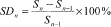or if a span adjustment was made on the previous test day,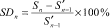where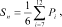n indicates the n-th test day, and i indicates the i-th measurement reading on the n-th test day.

(B) SD must be within the span drift limits (inclusive) specified in table B-1 to subpart B of part 53 to pass the test for span drift.

(iii) Lag time. Determine, from the strip chart (or alternative test data record), the elapsed time in minutes between the change in test concentration (or mark) made in step (xxvi) and the first observable (two times the noise level) measurement response. This time must be equal to or less than the lag time limit specified in table B-1 to subpart B of part 53 to pass the test for lag time.

(iv) Rise time. Calculate 95 percent of measurement reading P9 and determine, from the recorder chart (or alternative test data record), the elapsed time between the first observable (two times noise level) measurement response and a response equal to 95 percent of the P9 reading. This time must be equal to or less than the rise time limit specified in table B-1 to subpart B of part 53 to pass the test for rise time.

(v) Fall time. Calculate five percent of (P10 − L2) and determine, from the strip chart (or alternative test record), the elapsed time in minutes between the first observable decrease in measurement response following reading P10 and a response equal to L2 + five percent of (P10 − L2). This time must be equal to or less than the fall time limit specification in table B-1 to subpart B of part 53 to pass the test for fall time.

(vi) Precision. Calculate precision (both P20 and P80) for each test day as follows:

(A)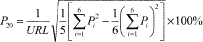(B)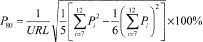(C) Both P20 and P80 must be equal to or less than the precision limits specified in table B-1 to subpart B of part 53 to pass the test for precision.

[76 FR 54326, Aug. 31, 2011, as amended at 80 FR 65460, Oct. 26, 2015]